Next: Existence of parameterized sets Up: Making Robots Conscious Previous: Relative consistency

# Inferring Non-knowledge

[This section and the next have a lot of redundancy. This will be fixed.]

Let p be a proposition. The proposition that the robot does not know p will be written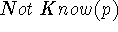, and we are interested in those mental situations s in which we have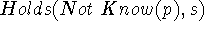. If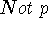is consistent with the robot's knowledge, then we certainly want.

How can we assert that the proposition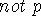is consistent with the robot's knowledge? Gödel's theorem tells us that we aren't going to do it by a formal proof using the robot's knowledge as axioms.The most perfunctory approach is for a program to try to prove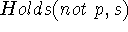from the robot's knowledge and fail. Logic programming with negation as failure does this for Horn theories.

However, we can often do better. If a person or a robot regards a certain collection of facts as all that are relevant, it suffices to find a model of these facts in which p is false.Consider asserting ignorance of the value of a numerical parameter. The simplest thing is to say that there are at least two values it could have, and therefore the robot doesn't know what it is. However, we often want more, e.g. to assert that the robot knows nothing of its value. Then we must assert that the parameter could have any value, i.e. for each possible value there are models of the relevant facts in which it has that value. Of course, complete ignorance of the values of two parameters requires that there be a model in which each pair of values is taken.

It is likely to be convenient in constructing these models to assume that arithmetic is consistent, i.e. that there are models of arithmetic. Then the set of natural numbers, or equivalently Lisp S-expressions, can be used to construct the desired models. The larger the robot's collection of theories postulated to have models, the easier it will be to show ignorance.

Making a program that reasons about models of its knowledge looks difficult, although it may turn out to be necessary in the long run. The notion of transcending a context may be suitable for this.

For now it seems more straightforward to use second order logic. The idea is to write the axioms of the theory with predicate and function variables and to use existential statements to assert the existence of models. Here's a proposal.

Suppose the robot has some knowledge expressed as an axiomatic theory and it needs to infer that it cannot infer that President Clinton is sitting down. We immediately have a problem with Gödel's incompleteness theorem, because if the theory is inconsistent, then every sentence is inferrable, and therefore a proof of non-inferrability of any sentence implies consistency. We get around this by using another idea of Gödel's--relative consistency.For example, suppose we have a first order theory with predicate symbols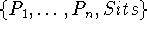and let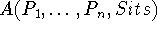be an axiom for the theory. The second order sentence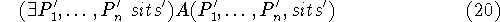expresses the consistency of the theory, and the sentence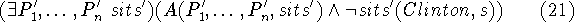expresses the consistency of the theory with the added assertion that Clinton is not sitting in the situation s. [In the above, we use upper case of the predicate constant Sits and lower case for the variable sits'.

Then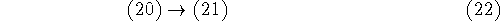is then the required assertion of relative consistency.

Sometimes we will want to assert relative consistency under fixed interpretations of some of the predicate symbols. This would be important when we have axioms involving these predicates but do not have formulas for them, e.g. of the form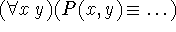. Suppose, for example, that there are three predicate symbols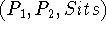, and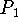has a fixed interpretation, and the other two are to be chosen so as to satisfy the axiom. Then the assertion of consistency with Clinton sitting takes the form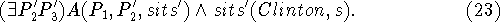The straightforward way of proving (23) is to find substitutions for the predicate variables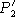and sits' that make the matrix of (23) true. The most trivial case of this would be when the axiom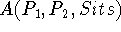does not actually involve the predicate Sits, and we already have an interpretation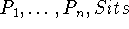in which it is satisfied. Then we can define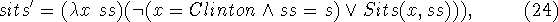and (23) follows immediately. This just means that if the new predicate does not interact with what is already known, then the values for which it is true can be assigned arbitrarily.Next: Existence of parameterized sets Up: Making Robots Conscious Previous: Relative consistency

John McCarthy
Mon Jul 15 13:06:22 PDT 2002